Chapter 10 Residual-based Item Fit Statistics

In this session, we will learn about
- residual-based item fit statistics
- simulation of item responses in R.

We have not launched into the topic of item fit until now, for a good reason. While it is a necessary step to check whether our item response data fit the IRT model we run, the residual-based item fit statistics have often been mis-interpreted, leading to quality items being discarded. It will be better not to use fit statistics than drawing wrong conclusions about the items. So if residual-based fit statistics are used, it is important to understand the properties of these statistics.

10.1 Redisual-based item fit statistics

Let $$X_{ni}$$ be the observed response of person $$n$$ on item $$i$$. $$X_{ni}$$ is a binomial random variable taking values of 0 and 1. The expected value of $$X_{ni}$$ is $$E_{ni}=p$$ (see Eq.(3.1) from the Rasch model), and the variance of $$X_{ni}$$ is $$W_{ni}=p(1-p)$$.

For each person $$n$$ and each item $$i$$, Wright and Masters (1982) defined a standardised residual statistic as

$\begin{equation} z_{ni} = \frac{x_{ni}-E_{ni}}{\sqrt{W_{ni}}}\tag{10.1} \end{equation}$

$$z_{ni}$$ looks very much like a $$z$$-score, except that $$x_{ni}$$ is a discrete variable (0 or 1), not a continuous variable. Nevertheless, $$z_{ni}$$ has distributional properties similar to those of a $$z$$-score.

Wright and Masters (1982) further defined a residual-based fit statistic as

$\begin{equation} u_i = \frac{ \sum\limits_{n=1}^{N} z_{ni}^2 }{N}\tag{10.2} \end{equation}$

where $$N$$ is the total number of students. $$u_i$$ is called unweighted fit mean square, or outfit. The term “outfit” calls attention to the fact that this statistic is sensitive to outliers. If there is an unexpected item response, such as an able student obtaining an incorrect answer on an easy item, or a low ability student correctly answering a difficult item, the fit mean square will tend to have large values. So an item may be deemed misfitting because there are some outliers by chance. To address this issue of the sensitivity to outliers, a weighted fit mean square is constructed as follows:

$\begin{equation} v_i = \frac{ \sum\limits_{n=1}^{N} W_{ni}z_{ni}^2 }{\sum\limits_{n=1}^{N} W_{ni}}\tag{10.3} \end{equation}$

The weight $$W_{ni}$$ in Eq.(10.3) is the variance of $$X_{ni}$$. $$W_{ni}$$ is large when the ability of a student matches the difficulty of an item. $$W_{ni}$$ is small when ability measure and item difficulty are far apart. $$v_i$$ is called weighted fit mean square, or infit for “information weighted fit” since $$W_{ni}$$ is also called the information function.

10.2 Critical values for fit mean square (fit MS) statistics

To be able to use the fit statistics to assess whether the item responses fit a model, we need to know the range of the values of the fit statistics when item responses do fit the model. We can derive these critical values either from statistical theory or from simulation. Below we run some simulations to find the distributional properties of the fit statistics when the item responses fit the Rasch model. We generate item responses fitting the Rasch model, with 2000 students whose abilities are drawn from N(0,1), and 40 items with difficulties from -2 to 2 at equal increments. The outfit MS values are computed for each item, and the simulation is replicated 100 times. The result of this simulation contains 100 outfit MS values for each item. The mean across the 100 replications is calculated for each item, as well as the standard deviation across the 100 outfit values.

library(TAM)
generateRasch <- function(N,I){
theta <- rnorm( N ) # student abilities
p1 <- plogis( outer( theta , seq( -2 , 2 , len=I ) , "-" ) )  #item difficulties from -2 to 2
resp <- 1 * ( p1 > matrix( runif( N*I ) , nrow=N , ncol=I ) )  # item responses
colnames(resp) <- paste("I" , 1:I, sep="")
return(list(resp=resp))
}

simulateFit <- function(N,I,Nrep){
outfit <- matrix(0,ncol=I,nrow=Nrep)
colnames(outfit) <- paste0("Item",seq(1,I))
for (r in 1:Nrep){
d <- generateRasch(N,I)
mod1 <- tam.jml(d$resp,bias=FALSE) fit1 <- tam.jml.fit(mod1,trim_val = NULL) outfit[r,] <- fit1$fit.item$outfitItem } return (list(outfitMS=outfit)) } set.seed(26473) N <- 2000 I <- 40 Nrep <- 100 s <- simulateFit(N,I,Nrep) apply(s$outfitMS,2,mean)
apply(s$outfitMS,2,sd) The result of the above simulation shows that the mean value of the outfit MS statistic is very close to 1, while the standard deviation ranges from 0.0251 to 0.0744, with an average of 0.0426. So, when the item response data fit the Rasch model, 95% of the outfit MS values will likely lie between 0.92 and 1.08, when the sample size is 2000. The following dot chart shows the spread of the outfit MS values for the 40 items for one replication (replication 2) (Figure 10.1). dotchart(s$outfitMS[2,], xlim=c(0.5,1.5), pt.cex=1, cex=0.5)
abline(v=0.9,lty=3)
abline(v=1.1,lty=3)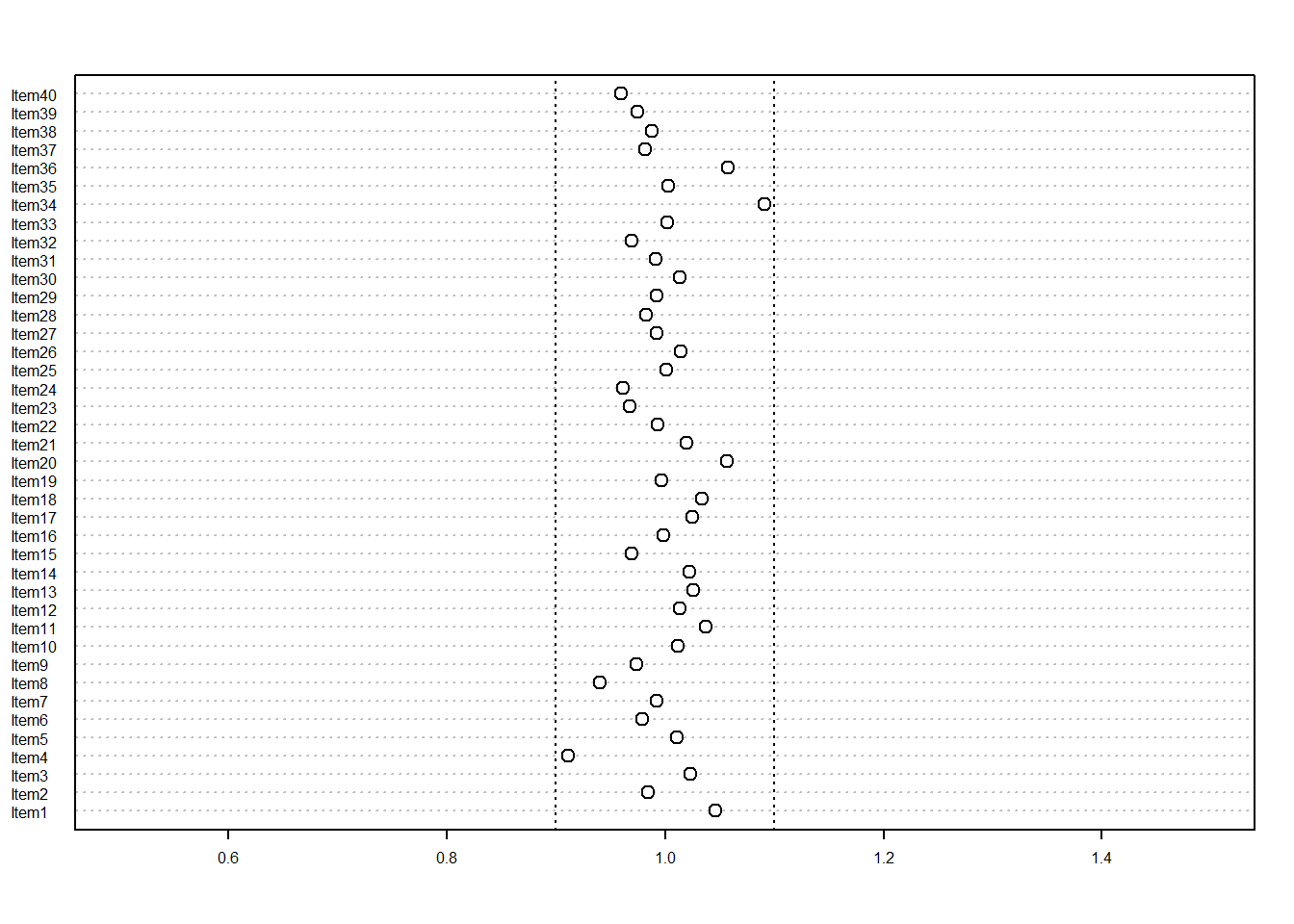Figure 10.1: outfit MS for sample size 2000

However, when we repeat the simulation for a sample size of 500 students, the spread of the outfit MS values is wider, as shown in Figure 10.2.

set.seed(4625)
N <- 500
I <- 40
Nrep <- 100
s <- simulateFit(N,I,Nrep)
dotchart(s$outfitMS[2,], xlim=c(0.5,1.5), pt.cex=1, cex=0.5) abline(v=0.8,lty=3) abline(v=1.2,lty=3)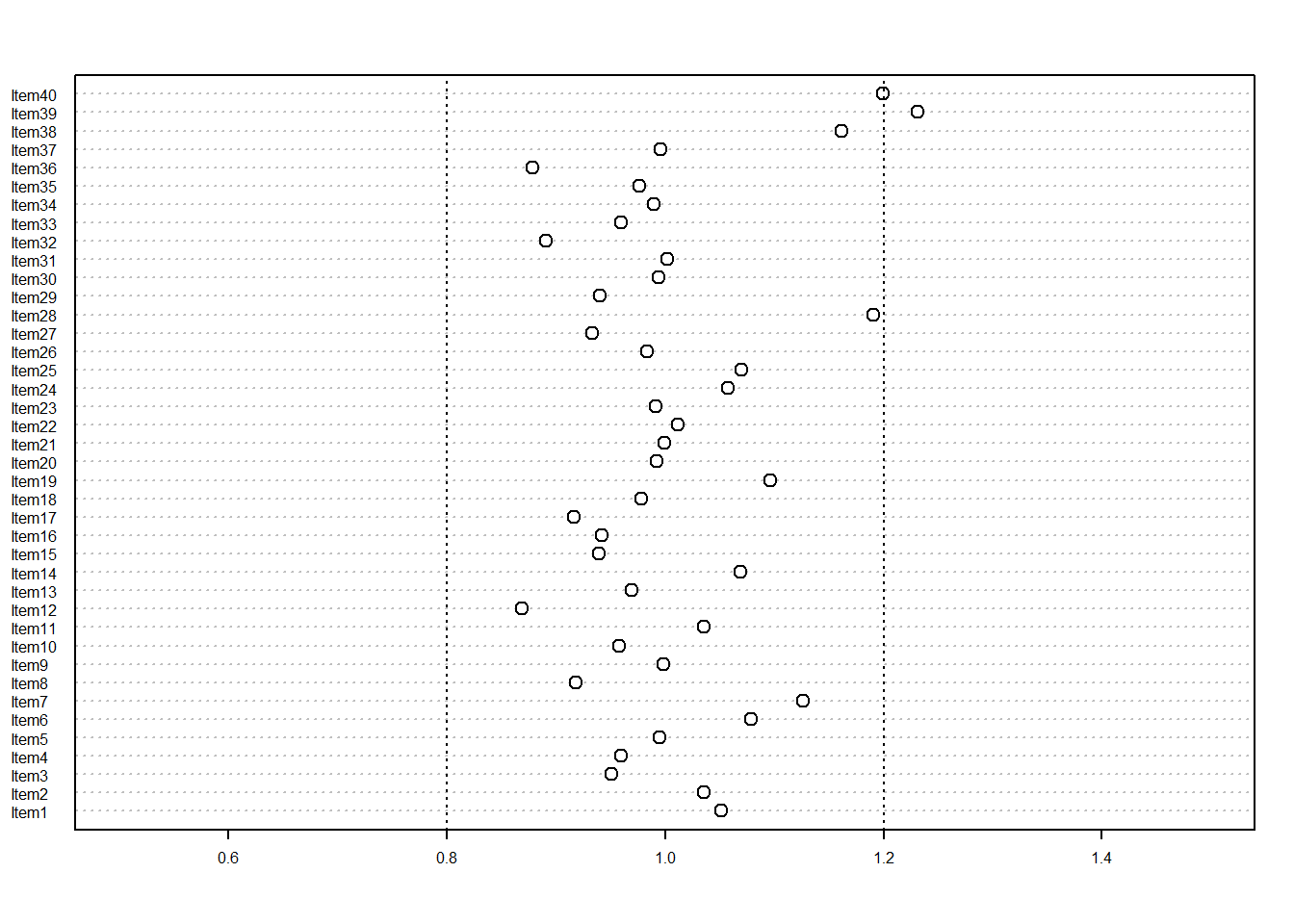Figure 10.2: outfit MS for sample size 500 It is apparent that the critical values for accepting items as fitting the Rasch model are dependent on the sample size of students. The range of fit MS values is large when sample size is small. In contrast, when the sample size is large (say, over 5000), the fit MS values are all very close to 1. Consequently, it becomes difficult to set critical values for identifying misfitting items, since we need to take sample size into account. One simple formula as an approximation to set the critical values is to use $\begin{equation} Asymptotic\: standard\: error\: for\: outfit\: MS = \sqrt {\frac{2}{N}}\tag{10.4} \end{equation}$ where $$N$$ is the sample size of students. As an example, when sample size is 2000, the asymptotic standard error is 0.032, so the range for accepting items as fitting the model is 0.94 and 1.06. In contrast, when sample size is 500, the asymptotic standard error is 0.063, so the range for accepting items as fitting the model is 0.87 and 1.13. Additional notes on the treatment of outliers in TAM In TAM, the function for calculating the residual-based fit statistics has an argument for trimming outliers. By default, the argument, trim_val is set to 10. Whenever a squared standardised residual is larger than trim_val, it is set to trim_val. We recommend to include this option so there won’t be excessively high values of fit MS due to an occasional outlier by chance. However, in the above R code for examining the distributional properties of the fit MS, we have turned this option off. 10.3 Infit or Outfit? Different researchers have made different recommendations regarding whether outfit or infit should be used. As we mentioned, outfit is likely to be impacted by the occasional outliers, leading to excessively high fit MS values by chance. Our recommendation is that infit is preferable to outfit so we do not inadvertently classify an item as misfitting due to chance. Although we will likely to be on the more conservative side of deciding making. 10.4 Two types of misfit: underfit and overfit When items fit the Rasch model, the fit MS is around the value of 1. Misfitting items may have fit MS values much lower than 1, or much larger than 1. In the case where the fit MS values are much larger than 1, the items are said to underfit. In the case where the fit MS values are much smaller than 1, the items are said to overfit. Before we discuss about underfit and overfit, we will first explain about the kind of misfit that the fit MS statistics can detect (and cannot detect). A frequent misunderstanding of misfitting items (identified by fit MS statistics) is that the theoretical ICC and observed ICC do not coincide. An example is shown in Figure 10.3 where the ICC of Item 4 in the CTTdata set is shown. library(TAM) library(CTT) data(CTTdata) data(CTTkey) CTTresp <- score(CTTdata, CTTkey, output.scored = TRUE) IA <- itemAnalysis(CTTresp$scored)

mod2 <- tam.jml(CTTrespscored) fit2 <- tam.jml.fit(mod2) plot(mod2,items=4)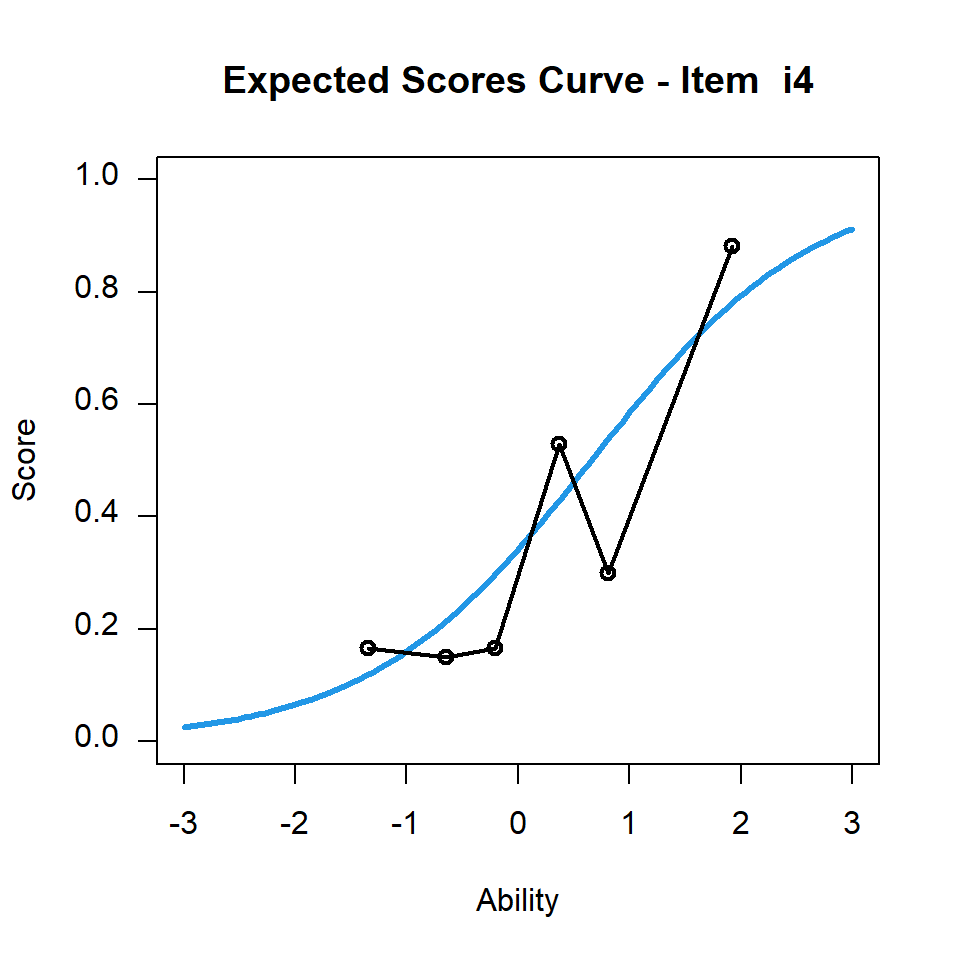Figure 10.3: CTTdata Item 4 Item Characteristic Curve The theoretical ICC and the observed ICC in Figure 10.3 do not seem to align at all. Yet the infit MS is 0.924. Figure 10.4 shows the ICC for the same item, however, for the observed ICC, students are grouped into four ability groups instead of six ability groups as in Figure 10.3. plot(mod2,items=4,ngroups=4)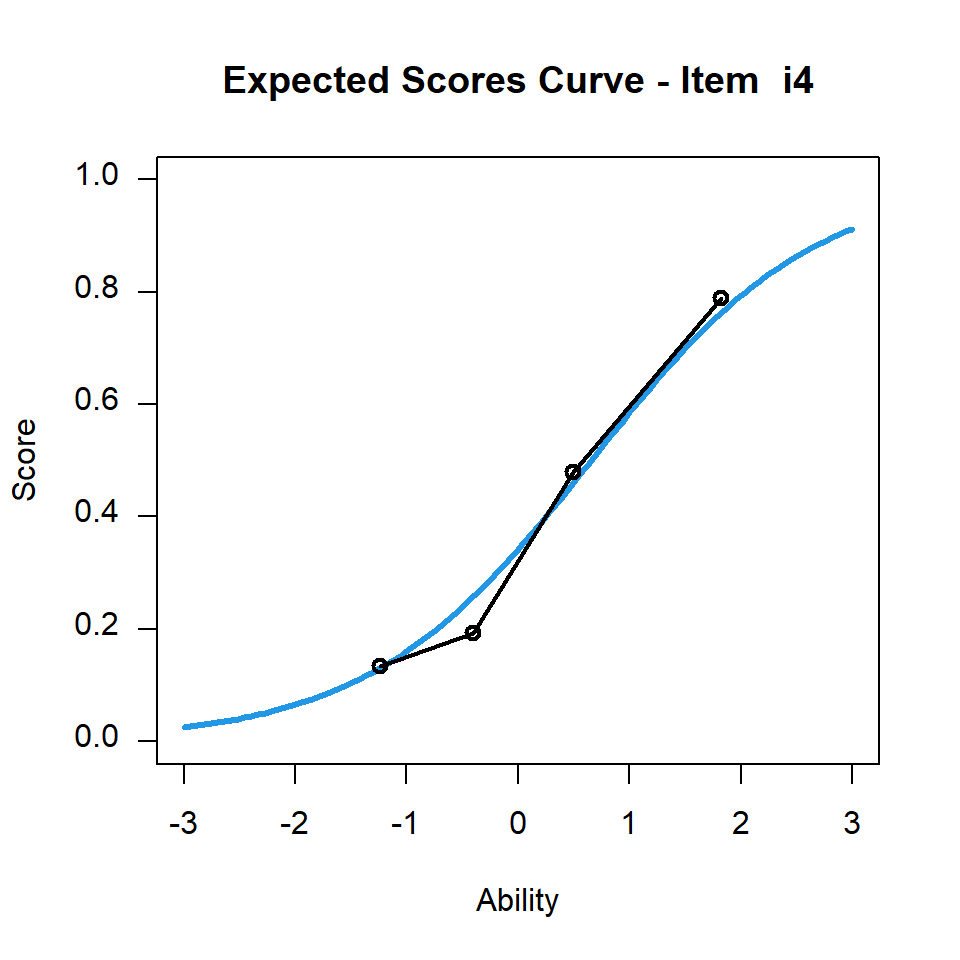Figure 10.4: CTTdata Item 4 Item Characteristic Curve Figure 10.4 shows that the observed ICC is very close to the theoretical ICC. As the data set CTTdata has only 100 students in total, dividing the students into more groups means that there are fewer students in each group, leading to quite fluctuating average scores for the groups. When we divide the students into only four groups, there are more students in each group, and the observed mean scores become closer to the theoretical mean scores. Consequently, a visual examination of the comparison between theoretical and observed ICCs does not provide a good indication of item fit. In general, it will be better to use fewer groups than many groups in plotting the observed ICC. For the CTTdata, the fit statistics are shown in Table 10.1. library(knitr) kable(fit2fit.item,digits=3,align="ccccc",caption="Item fit statistics for CTTdata",row.names=FALSE)
Table 10.1: Item fit statistics for CTTdata
item outfitItem outfitItem_t infitItem infitItem_t
i1 0.741 -1.621 0.835 -1.831
i2 0.823 -0.975 0.887 -1.280
i3 1.114 0.642 1.173 1.876
i4 1.037 0.264 0.924 -0.671
i5 0.958 -0.180 1.029 0.297
i6 0.754 -0.507 0.977 -0.105
i7 0.651 -1.312 0.816 -1.895
i8 0.937 -0.261 1.007 0.114
i9 1.100 0.535 1.098 0.808
i10 1.031 0.233 1.123 1.343
i11 0.798 -1.206 0.869 -1.295
i12 0.836 -0.796 0.801 -2.398
i13 1.142 0.686 1.068 0.778
i14 0.773 -1.316 0.844 -1.801
i15 1.235 1.305 1.152 1.392
i16 1.438 2.024 1.316 2.451
i17 0.883 -0.657 0.920 -0.771
i18 0.960 -0.137 0.984 -0.092
i19 1.207 1.074 1.052 0.474
i20 1.082 0.522 0.968 -0.281

What the fit MS detect is whether the observed ICC is steeper or flatter than the theoretical ICC. See . We will look at infit values instead of outfit values for the following discussions. Item 16 in Table 10.1 has an infit MS great than 1. Figure 10.5 shows the ICC.

plot(mod2,items=16,ngroups=3)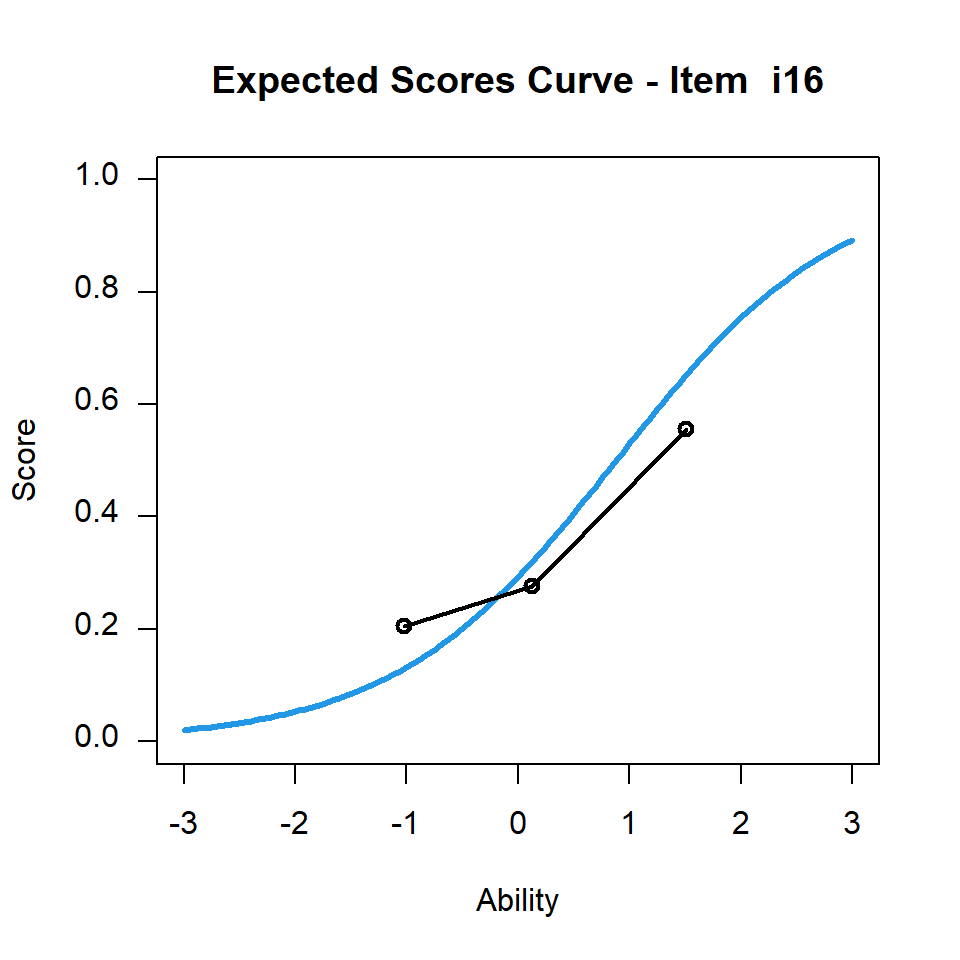Figure 10.5: CTTdata Item 16 Item Characteristic Curve

In contrast, Item 12 has an infit MS lower than 1, Figure 10.6 shows the ICC.

plot(mod2,items=12,ngroups=3)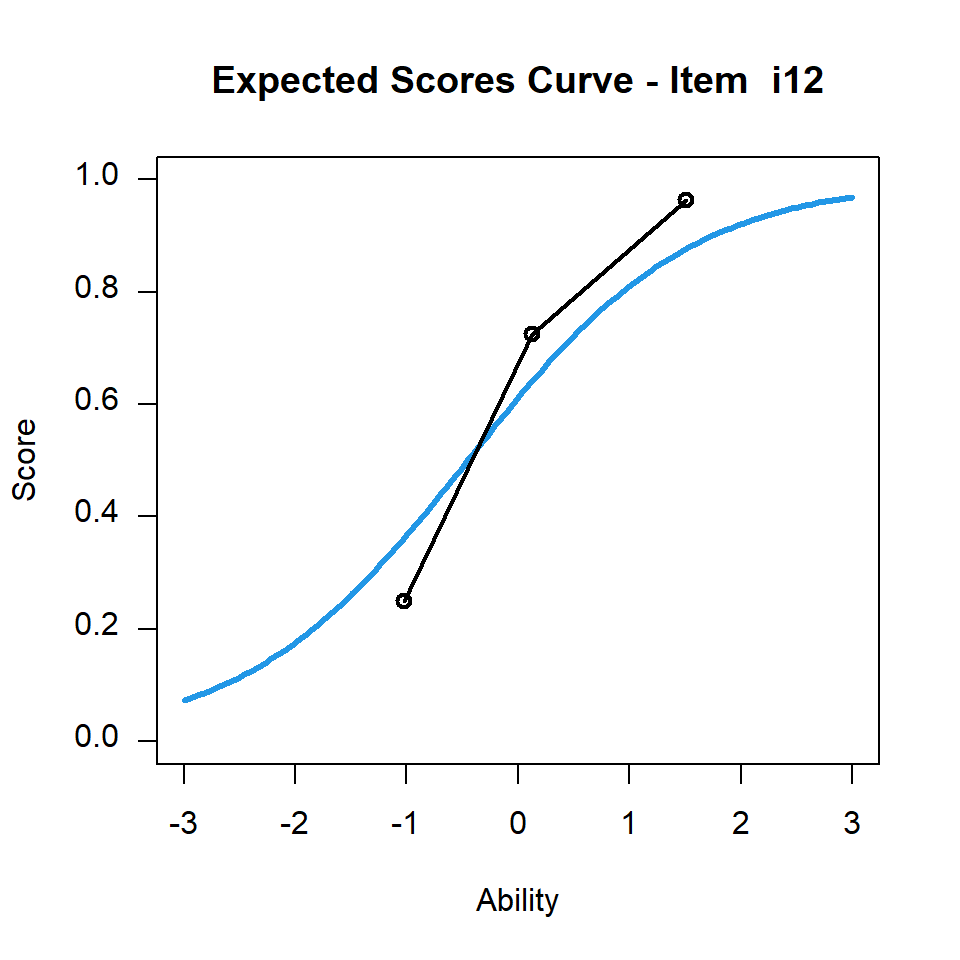Figure 10.6: CTTdata Item 12 Item Characteristic Curve

10.5 Residual-based fit statistics reflect item discrimination

The residual-based fit statistics reflect the slope of the ICC:
- When fit MS is close to 1, the item has average item discrimination of the set of items.
- When fit MS is lower than 1, the item is more discriminating than the average item discrimination.
- When fit MS is higher than 1, the item is less discriminating than the average item discrimination.

Consequently, high quality items are those with fit MS less than 1, even though some of these items may be deemed as misfitting the model, as their item discrimination is higher than the average. The items with fit MS high than 1 are poorer items as they do not discriminate students as well as other items. And finally, the items that are deemed fitting the model (with fit MS close to 1) are mediocre items, since their discrimination power is average.

A common mistake is to eliminate items with fit MS both higher and lower than 1, and retaining only items with fit MS close to 1. In this way, the best items are unfortunately eliminated.

10.6 Fit MS is relative

Fit is a relative statistic, as the values are relative to the fit values of the whole item set. If a subset of items is chosen, then the items with infit MS close to 1 will change. For example, let us choose only items with infit MS less than 1 and re-run the analysis.

resp3 <- CTTresp$scored[,fit2$fit.item[,4]<1]
colnames(resp3) <- paste("Item",which(fit2$fit.item[,4]<1)) mod3 <- tam.jml(resp3) fit3 <- tam.jml.fit(mod3) kable(fit3$fit.item,digits=3,align="ccccc",caption="Item fit statistics for rescaled CTTdata items where fit MS were less than 1",row.names=FALSE)
Table 10.2: Item fit statistics for rescaled CTTdata items where fit MS were less than 1
item outfitItem outfitItem_t infitItem infitItem_t
Item 1 0.888 -0.407 0.903 -0.812
Item 2 1.001 0.091 0.974 -0.202
Item 4 1.040 0.241 1.001 0.054
Item 6 0.702 -0.386 0.988 -0.040
Item 7 0.679 -0.786 0.885 -1.066
Item 11 0.962 -0.080 1.004 0.074
Item 12 0.718 -1.018 0.826 -1.694
Item 14 0.827 -0.646 0.918 -0.716
Item 17 0.822 -0.708 0.974 -0.166
Item 18 1.096 0.427 1.171 1.221
Item 20 1.108 0.512 1.044 0.386

The infit MS values in Table 10.2 for the subset of items where their infit MS were less than 1 in Table 10.1 are now centred around 1.

If we select only items with infit MS greater than 1 in Table 10.1 and re-run the analysis, we see that in Table 10.3 the infit MS values are all centred around 1 now.

resp4 <- CTTresp$scored[,fit2$fit.item[,4]>1]
colnames(resp4) <- paste("Item",which(fit2$fit.item[,4]>1)) mod4 <- tam.jml(resp4) fit4 <- tam.jml.fit(mod4) kable(fit4$fit.item,digits=3,align="ccccc",caption="Item fit statistics for rescaled CTTdata items where fit MS were greater than 1",row.names=FALSE)
Table 10.3: Item fit statistics for rescaled CTTdata items where fit MS were greater than 1
item outfitItem outfitItem_t infitItem infitItem_t
Item 3 1.041 0.303 1.086 1.109
Item 5 0.797 -1.323 0.889 -1.209
Item 8 0.909 -0.511 0.961 -0.489
Item 9 0.811 -1.038 0.881 -1.084
Item 10 0.900 -0.619 0.964 -0.448
Item 13 0.960 -0.160 1.014 0.202
Item 15 1.126 0.827 1.088 0.968
Item 16 1.058 0.386 1.107 1.019
Item 19 0.840 -0.933 0.859 -1.409

While the rescaled fit MS values are all centred around 1 whether we rescale a subset of items where all fit MS values were great than 1, or were all less than 1, there is one very big difference. The test reliability is vastly different. In the case of the subset of items where fit MS were all less than 1, the rescaled test reliability is 0.691. In contrast, the rescaled test reliability for the subset of items where the fit MS were all greater than 1 is 0.347. This is an important reason for why we should not remove items with fit MS values less than 1. The test reliability for the whole set of items in Table 10.1 is 0.779.

10.7 Fit t statistics

In Tables 10.1, 10.2, 10.3, there are two columns of output headed “outfitItem_t” and “infitItem_t.” These are fit t-statistics. They are transformed fit MS values to a z-score. That is, the fit t-statistics can be treated as a N(0,1) variable. If the values are outside (-2, 2), one can conclude that the fit MS value is statistically significantly different from 1. In transforming the fit MS values to a z-score, the sample size of students, $$N$$, is taken into account. So fit t-statistics can be interpreted as a N(0,1) variable regardless of the sample size. This is useful since the critical values of fit MS statistics vary depending on the sample size. For fit-t statistics, we just assess whether the values are within (-2, 2). This, seemingly, is a good solution to the problem of varying critical values of the fit MS statistics. However, in practice, there are also issues. This relates (again) to the difference between theory and practice.

10.8 Real data sets versus simulated data sets

When we simulate data sets according to the Rasch model, we can use theoretical distributional properties and significance tests as we have described. In real-life though, item response data sets rarely fit the Rasch model with equal discrimination between items. In our experience, we are yet to find a single (real) data set that fits the Rasch model. Items have inherently different discrimination power. Unlike item difficulty which test writers can largely control, few test writers can predict the discrimination power of an item, let alone write items with fixed discrimination. To use the Rasch model, we need to tolerate differing item discrimination. But statistically, if the sample size of students is sufficiently large, nearly all test items will have statistically significant fit-t values, simply because that is the “truth” (that items differ in discrimination) that can be picked up with a large sample.

An example is given below. The PISA 2012 Mathematics Booklet 10 scored item responses can be downloaded here. The following R code reads in the data file, scales the data with tam.jml and computes residual-based item fit statistics.

rm(list=ls())
library(TAM)
setwd("C:\\G_MWU\\ARC\\Philippines\\files")
mod5 <- tam.jml(resp)
fit5 <- tam.jml.fit(mod5)
kable(fit5fit.item,digits=3,align="ccccc",caption="Item fit statistics for PISA 2012 Math Booklet 10",row.names=FALSE) Table 10.4: Item fit statistics for PISA 2012 Math Booklet 10 item outfitItem outfitItem_t infitItem infitItem_t PM00KQ02 0.868 -5.001 0.945 -5.328 PM033Q01 1.095 6.724 1.050 7.991 PM034Q01T 0.897 -7.848 0.957 -6.525 PM155Q01 0.861 -14.248 0.887 -21.499 PM155Q02D 1.121 6.836 1.080 10.272 PM155Q03D 0.704 -11.043 0.933 -6.018 PM155Q04T 1.041 3.970 1.030 5.375 PM273Q01T 1.188 17.986 1.136 24.156 PM408Q01T 1.011 0.887 1.037 6.011 PM411Q01 0.788 -20.635 0.873 -22.842 PM411Q02 1.179 15.662 1.095 15.883 PM420Q01T 1.120 11.534 1.082 14.725 PM442Q02 0.721 -21.044 0.841 -24.614 PM446Q01 0.857 -13.480 0.885 -21.567 PM446Q02 0.444 -14.981 0.804 -12.267 PM447Q01 0.936 -5.768 0.977 -4.220 PM462Q01D 0.813 -5.042 0.989 -0.837 PM464Q01T 0.629 -22.206 0.818 -24.482 PM474Q01 1.161 13.325 1.057 9.838 PM559Q01 1.141 11.589 1.102 17.498 PM800Q01 1.424 10.933 1.072 5.626 PM803Q01T 0.630 -22.548 0.822 -23.740 PM828Q01 0.857 -10.531 0.958 -6.519 PM828Q02 1.048 4.612 1.019 3.489 PM828Q03 1.020 1.360 1.076 11.175 PM906Q01 0.994 -0.575 1.008 1.578 PM906Q02 0.862 -7.499 0.951 -6.365 PM915Q01 1.202 15.891 1.050 8.283 PM915Q02 0.916 -6.920 0.920 -14.094 PM982Q01 1.245 8.187 1.012 1.169 PM982Q02 1.125 9.119 1.125 19.168 PM982Q03T 1.194 15.448 1.088 15.089 PM982Q04 0.963 -3.810 0.936 -12.098 PM992Q01 0.922 -4.938 1.002 0.326 PM992Q02 0.853 -7.097 0.916 -10.021 PM992Q03 0.421 -18.975 0.766 -17.681 Almost all of the infit t-statistics in Table 10.4 are outside the range of (-2, 2). This is because the sample size (35421) is very large, providing the power to detect small deviations of the fit MS values from 1. A random sample of 1000 students is selected from the file “PISA2012MathBk10.csv,” and the selected sample is rescaled. sample1000 <- sample(seq(1:nrow(resp)), 1000) resp1000 <- resp[sample1000, ] mod6 <- tam.jml(resp1000) fit6 <- tam.jml.fit(mod6) kable(fit6fit.item,digits=3,align="ccccc",caption="Item fit statistics for PISA 2012 Math Sample of 1000 Students",row.names=FALSE)
Table 10.5: Item fit statistics for PISA 2012 Math Sample of 1000 Students
item outfitItem outfitItem_t infitItem infitItem_t
PM00KQ02 0.860 -0.847 0.914 -1.404
PM033Q01 0.998 0.005 1.015 0.409
PM034Q01T 0.969 -0.350 1.019 0.494
PM155Q01 0.830 -3.026 0.879 -3.826
PM155Q02D 1.133 1.346 1.111 2.407
PM155Q03D 0.629 -2.208 0.897 -1.492
PM155Q04T 1.067 1.140 1.044 1.345
PM273Q01T 1.201 3.342 1.131 3.934
PM408Q01T 1.069 0.915 1.074 2.014
PM411Q01 0.876 -1.953 0.911 -2.677
PM411Q02 1.222 3.435 1.106 3.087
PM420Q01T 1.123 2.089 1.073 2.235
PM442Q02 0.718 -3.586 0.833 -4.440
PM446Q01 0.884 -1.817 0.921 -2.341
PM446Q02 0.435 -2.484 0.793 -2.159
PM447Q01 0.976 -0.328 1.010 0.289
PM462Q01D 0.695 -1.287 0.914 -1.082
PM464Q01T 0.662 -3.448 0.851 -3.408
PM474Q01 1.189 2.876 1.056 1.638
PM559Q01 1.106 1.597 1.112 3.165
PM800Q01 1.206 0.968 1.082 1.017
PM803Q01T 0.626 -3.445 0.810 -4.049
PM828Q01 0.881 -1.454 0.972 -0.703
PM828Q02 0.983 -0.274 0.953 -1.454
PM828Q03 1.012 0.178 1.133 3.361
PM906Q01 0.960 -0.671 0.978 -0.658
PM906Q02 0.877 -1.084 0.983 -0.356
PM915Q01 1.224 2.979 1.018 0.524
PM915Q02 0.879 -1.714 0.927 -2.067
PM982Q01 1.166 1.036 0.959 -0.659
PM982Q02 1.129 1.655 1.125 3.292
PM982Q03T 1.160 2.264 1.075 2.102
PM982Q04 1.069 1.209 0.990 -0.296
PM992Q01 0.701 -3.180 0.881 -2.782
PM992Q02 0.829 -1.532 0.932 -1.450
PM992Q03 0.413 -3.164 0.758 -3.045

Table 10.5 shows that the infit t-statistics are smaller than those in Table 10.4, although there are still many outside the range of (-2, 2).

Table 10.6 shows the infit t-statistics when a random sample of 200 students is selected.

sample200 <- sample(seq(1:nrow(resp)), 200)
resp200 <- resp[sample200, ]
mod7 <- tam.jml(resp200)
fit7 <- tam.jml.fit(mod7)
kable(fit7\$fit.item,digits=3,align="ccccc",caption="Item fit statistics for PISA 2012 Math Sample of 200 Students",row.names=FALSE)
Table 10.6: Item fit statistics for PISA 2012 Math Sample of 200 Students
item outfitItem outfitItem_t infitItem infitItem_t
PM00KQ02 1.095 0.410 1.053 0.428
PM033Q01 0.848 -0.924 0.971 -0.326
PM034Q01T 0.714 -2.066 0.829 -2.101
PM155Q01 0.753 -2.178 0.842 -2.335
PM155Q02D 0.935 -0.272 1.072 0.779
PM155Q03D 0.751 -0.592 0.886 -0.718
PM155Q04T 1.155 1.371 1.115 1.632
PM273Q01T 1.103 0.961 1.086 1.289
PM408Q01T 1.015 0.148 1.079 0.958
PM411Q01 0.877 -1.118 0.920 -1.170
PM411Q02 0.953 -0.357 0.959 -0.553
PM420Q01T 1.157 1.422 1.133 1.932
PM442Q02 0.770 -1.688 0.887 -1.412
PM446Q01 0.939 -0.341 0.862 -1.751
PM446Q02 0.496 -1.102 0.830 -0.763
PM447Q01 0.963 -0.258 1.006 0.115
PM462Q01D 1.472 1.115 1.082 0.531
PM464Q01T 0.406 -2.734 0.672 -2.883
PM474Q01 1.109 0.925 1.128 1.777
PM559Q01 1.130 0.896 1.115 1.456
PM800Q01 1.258 0.671 1.100 0.554
PM803Q01T 0.688 -1.642 0.888 -1.092
PM828Q01 0.686 -2.132 0.829 -2.100
PM828Q02 1.045 0.444 0.989 -0.142
PM828Q03 0.964 -0.252 0.979 -0.265
PM906Q01 0.894 -0.844 0.994 -0.063
PM906Q02 0.973 -0.027 1.045 0.481
PM915Q01 1.647 4.314 1.247 3.150
PM915Q02 0.820 -1.280 0.918 -1.070
PM982Q01 1.212 0.770 1.012 0.132
PM982Q02 1.141 1.039 1.167 2.092
PM982Q03T 1.282 1.817 1.057 0.742
PM982Q04 0.902 -0.910 0.910 -1.379
PM992Q01 0.942 -0.231 0.914 -0.900
PM992Q02 0.804 -0.866 0.995 -0.008
PM992Q03 0.722 -0.818 0.810 -1.311

Table 10.6 shows that only 6 items have a statistically significant infit t value (outside (-2, 2)), while the remaining 30 items show that they fit the Rasch model.

10.9 So how do we use residual-based fit statistics?

While in theory, it is important to check for model fit whenever a mathematical model is used to fit the data, the interpretations of the residual-based fit statistics are far from straightforward. The following is a summary of do’s and don’t’s.

• Check items with underfit (fit MS > 1), but do not remove items with overfit (fit MS < 1).
• For overfit items, check if the item scores (weights) should be increased. We will have more discussions on this in the chapters on partial credit items and two-parameter models.
• Use infit-t statistics for more conservative evaluations of the items, but be aware that a large sample can lead to very large fit-t values.
• Use the fit statistics in conjunction with other item statistics, in particular, point-biserial correlations.

10.10 Homework

Simulate an item response data set fitting the Rasch model, with 200 students whose abilities are drawn from N(0,1), and 30 items with item difficulties from -2 to 2. Fit the Rasch model, and compute residual-based fit statistics. What is the range of the outfit MS and infit MS values?

References

Wright, Benjamin, and Geofferey Masters. 1982. Rating Scale Analysis. Book. Mesa Press Chicago.
Wu, Margaret, and Raymond J. Adams. 2013. “Properties of Rasch Residual Fit Statistics.” Journal of Applied Measurement 14 (4): 339–55.